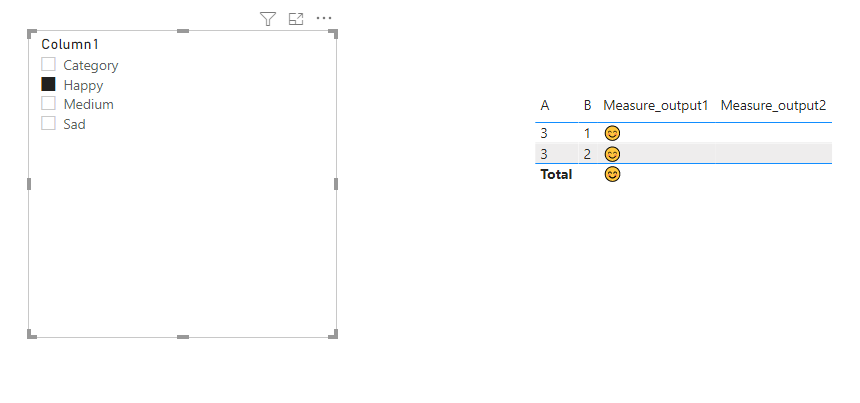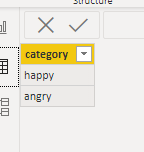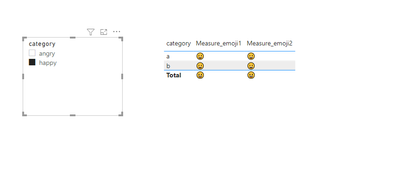cancel
Showing results for
Did you mean:

Fabric is Generally Available. Browse Fabric Presentations. Work towards your Fabric certification with the Cloud Skills Challenge.Helper IV

## Import excel table with dynamic images

Hey everybody,

I am an absolute beginner at PowerBi. I have made an excel table (would love to attach but forum wont let me), wherein you can select 3 values. Theses 3 values then translate to an image (smileys). This is displayed on another page in a table.

Is it possible to import this table (as is) into powerbi? When I import the excel file it just shows data.

1 ACCEPTED SOLUTIONCommunity Support

Hi @Irwin ,

Create 2 measures as below:

``````Measure_output1 =
IF(ISFILTERED(Table2[Column1]),
SWITCH(SELECTEDVALUE(Table2[Column1]),
"Happy",
CALCULATE(MAX('Table1'[Output 1]),FILTER('Table1','Table1'[Output 1]="😊")),
"Medium",
CALCULATE(MAX('Table1'[Output 1]),FILTER('Table1','Table1'[Output 1]="🤔")),
CALCULATE(MAX('Table1'[Output 1]),FILTER('Table1','Table1'[Output 1]="😢"))),
CALCULATE(MAX('Table1'[Output 1]),FILTER('Table1','Table1'[A]=SELECTEDVALUE(Table1[A]))))``````
``````Measure_output2 =
IF(ISFILTERED(Table2[Column1]),
SWITCH(SELECTEDVALUE(Table2[Column1]),
"Happy",
CALCULATE(MAX('Table1'[Output 2]),FILTER('Table1','Table1'[Output 2]="😊")),
"Medium",
CALCULATE(MAX('Table1'[Output 2]),FILTER('Table1','Table1'[Output 2]="🤔")),
CALCULATE(MAX('Table1'[Output 2]),FILTER('Table1','Table1'[Output 2]="😢"))),
CALCULATE(MAX('Table1'[Output 1]),FILTER('Table1','Table1'[A]=SELECTEDVALUE(Table1[A]))))``````

And you will see:For the related .pbix file,pls see attached.

Best Regards,
Kelly

11 REPLIES 11Helper IV

Thank you.

So this means I can still import the dynamic values (1,2,3) and then create the image display in PowerBi itself?Community Support

Hi  @Irwin ，

Not sure whether I understand correctly. Coud you pls attach some screenshots to make your requirement more clear?

Best Regards,
KellyHelper IV

Sure.

I think most of the problem stems from me being super new to all of this.
The below scren is what I have made in excel. When I change a value (sad/medium/happy... could as well be 1,2,3) the smiley changes correspondingly. I would like to be able to change the value (sad/medium/happy) in excel and  then the smiley table would import this value in PowerBi.I now realize that I need to create the value to image part inside powerbi, however, watching tutorials have not quite equiped me to do so yet on my own 😉
Any help is appreciated!Community Support

Hi @Irwin ,

First create dim table as below:Then create 2 measures as below:

``````Measure_emoji1 =
SWITCH(SELECTEDVALUE('dim table'[category]),
"happy",
CALCULATE(MAX('Table'[emoj 1]),FILTER('Table','Table'[emoj 1]=UNICHAR("128512"))),
"angry",
CALCULATE(MAX('Table'[emoj 1]),FILTER('Table','Table'[emoj 1]=UNICHAR("128520"))))``````
``````Measure_emoji2 =
SWITCH(SELECTEDVALUE('dim table'[category]),
"happy",
CALCULATE(MAX('Table'[emoj 2]),FILTER('Table','Table'[emoj 2]=UNICHAR("128512"))),
"angry",
CALCULATE(MAX('Table'[emoj 2]),FILTER('Table','Table'[emoj 2]=UNICHAR("128520"))))``````

And you will see:For the unichar of emoji,see below for reference:

https://powerbidocs.com/2020/06/08/how-to-use-emojis-emotions-on-power-bi/

My sample .pbix file is attached,you may turn to it if needed.

Best Regards,
KellyHelper IV

Hi Kelly,

I have now had proper time to read your excellent feedback and mess around with it myself. I think that the main reason I cannot (could not?) get this to work is my lack of basic understanding. I am very much trying to work on that! 🙂

I have now managed to contruct something that I am quite satisfied with:however, I cannot make it display correctly in the report. It just shows the first 5 rows.... why?Thank you for any assistance.Helper IV

Thank you so much for this! I have not yet managed to completly set it up as I want but I am really learning from you attached file. I will look into it more when time at work permits it.

Again really appreciate it. 🙂Community Support

Hi  @Irwin ,

It's because that my sample data structure is different from yours ,could you pls upload your .pbix file to onedrive business and share the link with us?

Remember to remove the confidential information.

Best Regards,
KellyHelper IV

Hi Kelly,

No worries. I am just thank full for the assistance. I believe you should be able to access the file in the below link.

https://arlafoods-my.sharepoint.com/:u:/p/amibr/EdflN7UW6rVOlS2nkgjqwUcB04yrwDKX47IjHMCsSsvKdA?e=sTK...Community Support

Hi @Irwin ,

Create 2 measures as below:

``````Measure_output1 =
IF(ISFILTERED(Table2[Column1]),
SWITCH(SELECTEDVALUE(Table2[Column1]),
"Happy",
CALCULATE(MAX('Table1'[Output 1]),FILTER('Table1','Table1'[Output 1]="😊")),
"Medium",
CALCULATE(MAX('Table1'[Output 1]),FILTER('Table1','Table1'[Output 1]="🤔")),
CALCULATE(MAX('Table1'[Output 1]),FILTER('Table1','Table1'[Output 1]="😢"))),
CALCULATE(MAX('Table1'[Output 1]),FILTER('Table1','Table1'[A]=SELECTEDVALUE(Table1[A]))))``````
``````Measure_output2 =
IF(ISFILTERED(Table2[Column1]),
SWITCH(SELECTEDVALUE(Table2[Column1]),
"Happy",
CALCULATE(MAX('Table1'[Output 2]),FILTER('Table1','Table1'[Output 2]="😊")),
"Medium",
CALCULATE(MAX('Table1'[Output 2]),FILTER('Table1','Table1'[Output 2]="🤔")),
CALCULATE(MAX('Table1'[Output 2]),FILTER('Table1','Table1'[Output 2]="😢"))),
CALCULATE(MAX('Table1'[Output 1]),FILTER('Table1','Table1'[A]=SELECTEDVALUE(Table1[A]))))``````

And you will see:For the related .pbix file,pls see attached.

Best Regards,
KellySuper User

@Irwin , You can import excel as data. Unichar and some other formats can be used to create images. You have a check that out

Unichar :Super User

@Irwin , Excel is only for data export. You can export ppt or pdf the display format.

https://docs.microsoft.com/en-us/power-bi/visuals/power-bi-visualization-export-dataAnnouncements#### Power BI Monthly Update - November 2023

Check out the November 2023 Power BI update to learn about new features.#### Fabric Community News unified experience

Read the latest Fabric Community announcements, including updates on Power BI, Synapse, Data Factory and Data Activator.#### The largest Power BI and Fabric virtual conference

130+ sessions, 130+ speakers, Product managers, MVPs, and experts. All about Power BI and Fabric. Attend online or watch the recordings.Top Solution Authors
Top Kudoed Authors
Users online (2,814)